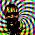Sunday, June 28, 2009

Burgers' Equation on a Moving Grid: Derivative Forcing

Solving Burgers' equation on a moving grid is a way to handle moving fronts or shocks by adapting the grid to the solution at each time-step. One simple monitor function is just the square of the solution derivative. Since we chose the forced diffusion equation as the governing equation for the grid, we just substitute the central difference approximation for the first derivative squared for the forcing function.We also include two parameters (s1, s2) so we can adjust the strength of the forcing compared to the smoothing (second derivative term). That gives our semi-discrete equation for the rates asHere's an example of the algorithm applied to an initial condition made-up of a couple exponential functions.It shows that the delta-x decreases in areas of high gradients and increases in areas of zero derivative. The wave gets diffused pretty significantly because of the backward Euler time integration scheme.

Another illustrative example of this adaptive grid scheme is shown below. The initial condition is a couple wavelengths of cosine. This example shows that we probably want to force grid points into areas of high curvature (second derivative) as well as high first derivative. Notice the large delta-x around the peaks of each wave, zero derivative in this area, but large second derivative.1.2.Dr Mitchell at NIST has a recent paper on a collection of test cases for adaptive grid methods in 2D (focused on elliptic problems, but the ideas are portable).

3.4.ADAPTIVE MESH REFINEMENT USING WAVE-PROPAGATION ALGORITHMS FOR HYPERBOLIC SYSTEMS [pdf]
Abstract:An adaptive mesh refinement algorithm developed for the Euler equations of gas dynamics has been extended to employ high-resolution wave-propagation algorithms in a more gen- eral framework. This allows its use on a variety of new problems, including hyperbolic equations not in conservation form, problems with source terms or capacity functions, and logically rectangular curvilinear grids. This framework requires a modified approach to maintaining consistency and conservation at grid interfaces, which is described in detail. The algorithm is implemented in the amrclaw package, which is freely available.
Keywords: adaptive mesh refinement, hyperbolic conservation laws, high resolution, Godunov, finite-volume methods, gas dynamics, acoustics, software

5.